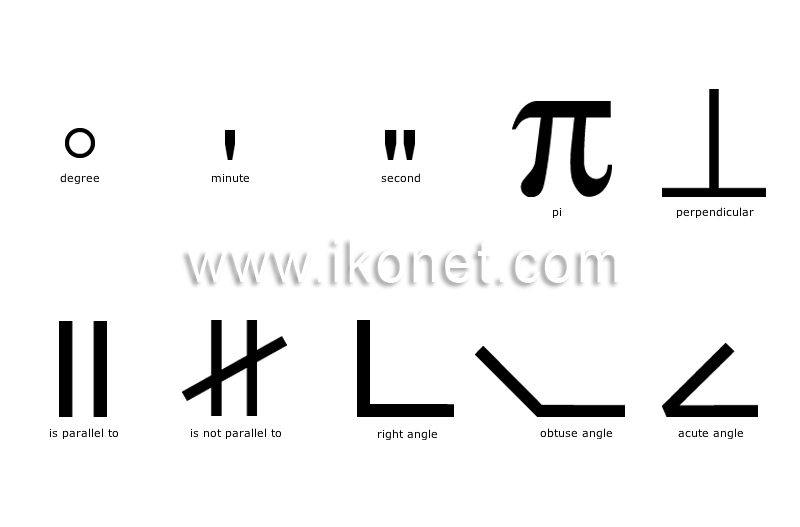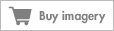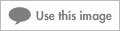Look up an image:

#### geometryMathematical discipline that studies the relations between points, straight lines, curves, surfaces and volumes.See geometry in : french | spanish

#### obtuse angleAngle between 90° and 180°.

#### acute angleAngle that is smaller than a right angle (less than 90°).

#### right angleAngle formed by two lines or two perpendicular planes that measures 90°.

#### perpendicularSymbol denoting that a straight line meets another at a right angle.

#### is not parallel toSymbol denoting that two straight lines do not remain at a constant distance from one other.

#### is parallel toSymbol denoting that two straight lines remain at a constant distance from one another.

#### minuteSymbol placed in superscript after a number that denotes degrees in sixtieths of a measure.

#### secondSymbol placed in superscript after a number that denotes degrees in sixtieths of a minute.

#### piConstant that represents the ratio of a circle’s circumference to its diameter; its value is approximately 3.1416.

#### degreeSymbol placed in superscript after a number to denote the opening of an angle or the length of an arc, or in front of an uppercase letter to identify a scale of measurement.